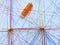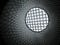# Leetcode Add One Row to Tree

Given the root of a binary tree, then value `v` and depth `d`, you need to add a row of nodes with value `v` at the given depth `d`. The root node is at depth 1.

The adding rule is: given a positive integer depth `d`, for each NOT null tree nodes `N` in depth `d-1`, create two tree nodes with value `v` as `N's` left subtree root and right subtree root. And `N's` original left subtree should be the left subtree of the new left subtree root, its original right subtree should be the right subtree of the new…

# Leetcode Longest Harmonious Sequence

We define a harmonious array as an array where the difference between its maximum value and its minimum value is exactly `1`.

Given an integer array `nums`, return the length of its longest harmonious subsequence among all its possible subsequences.

A subsequence of array is a sequence that can be derived from the array by deleting some or no elements without changing the order of the remaining elements.

## Constraints

• `1 <= nums.length <= 2 * 10^4`
• `-10^9 <= nums[i] <= 10^9`

## Difficulty

• Level: Easy
• Acceptance: 51.2%

## Analysis

For this question, I used a map just for the…

# Leetcode Buddy Strings

Given two strings `A` and `B` of lowercase letters, return `true` if you can swap two letters in `A` so the result is equal to `B`, otherwise, return `false`.

Swapping letters is defined as taking two indices `i` and `j` (0-indexed) such that `i != j` and swapping the characters at `A[i]` and `A[j]`. For example, swapping at indices `0` and `2` in `"abcd"` results in `"cbad"`.

## Constraints

• `0 <= A.length <= 20000`
• `0 <= B.length <= 20000`
• `A` and `B` consist of lowercase letters.

## Difficulty

• Level: Easy
• Acceptance: 29.5%

## Analysis

For this problem, one of the…

# Leetcode Linked List Cycle

Given `head`, the head of a linked list, determine if the linked list has a cycle in it.

There is a cycle in a linked list if there is some node in the list that can be reached again by continuously following the `next` pointer. Internally, `pos` is used to denote the index of the node that tail's `next` pointer is connected to. Note that `pos` is not passed as a parameter.

Return `true` if there is a cycle in the linked list. Otherwise, return `false`.

## Difficulty

• Level: Easy
• Acceptance: 42.6%

I used a set…

# Leetcode Trim a Binary Search Tree

Given the `root` of a binary search tree and the lowest and highest boundaries as `low` and `high`, trim the tree so that all its elements lies in `[low, high]`. Trimming the tree should not change the relative structure of the elements that will remain in the tree (i.e., any node's descendant should remain a descendant). It can be proven that there is a unique answer.

Return the root of the trimmed binary search tree. Note that the root may change depending on the given bounds.

## Difficulty

• Level: Medium
• Acceptance: 64.2%

## Analysis

I solved this problem…

# Leetcode Number of 1 Bits

Write a function that takes an unsigned integer and returns the number of ‘1’ bits it has (also known as the Hamming weight).

Note:

Note that in some languages such as Java, there is no unsigned integer type. In this case, the input will be given as a signed integer type. It should not affect your implementation, as the integer’s internal binary representation is the same, whether it is signed or unsigned.

In Java, the compiler represents the signed integers using 2’s complement notation. Therefore, in Example 3 above, the input represents the signed integer. `-3`.

Follow up: If this…

# Leetcode Vertical Order Traversal of a Binary Tree

Today, we will be looking at the following problem listed as hard with an acceptance rate of 38.6% at the time of writing.

The problem goes as follows:

Given the `root` of a binary tree, calculate the vertical order traversal of the binary tree.

For each node at position `(row, col)`, its left and right children will be at positions `(row + 1, col - 1)` and `(row + 1, col + 1)` respectively. The root of the tree is at `(0, 0)`.

The vertical order traversal of a binary tree is a list of top-to-bottom orderings for each column…

# Leetcode Concatenation of Consecutive Binary Numbers

Today, we will be looking at the following problem:

Given an integer `n`, return the decimal value of the binary string formed by concatenating the binary representations of `1` to `n` in order, modulo `10^9 + 7`.

Here is my solution:

In order to figure out this problem, I had to learn about bitwise operations and how shifting bits to the left is equal to multiplying the number by `2 * (number of places shifted)`.

For example, if I had the number `25` which translates to `11001`. A left shift would result in `110010` which is just a…

# How I became An AWS Certified Cloud Practitioner Within 6 DaysPhoto by Omar Flores on Unsplash

# Motivation For the CCPs

Before diving into the AWS Certified Cloud Practitioner or CCPs, let me tell you more about why “the cloud” excites me.

As you can see “the cloud” is everywhere around us. We can see that GitHub is a cloud repository for our code and services such as MongoDB Atlas or Heroku are helping you host your applications on the cloud. It’s easy to take for granted that we can very easily host those applications on storage that we do not own.

# Learning TypeScript — FunctionsPhoto by Rehan Syed on Unsplash

This is part 3 of the Learning TypeScript series. In part 2, we looked into what interfaces in TypeScript are and how they describe the shape of our objects in JavaScript. In this article, we will be exploring functions in more detail.

# Function Typing

TypeScript supports both named and anonymous functions just like in JavaScript. To add types to our functions, we could explicitly add types to our parameters and return values (optional).

Another way we can type check is by defining the type first before referencing that type with our function.

If you have been keeping up with this…## Bryan W.

I have a passion for coding.